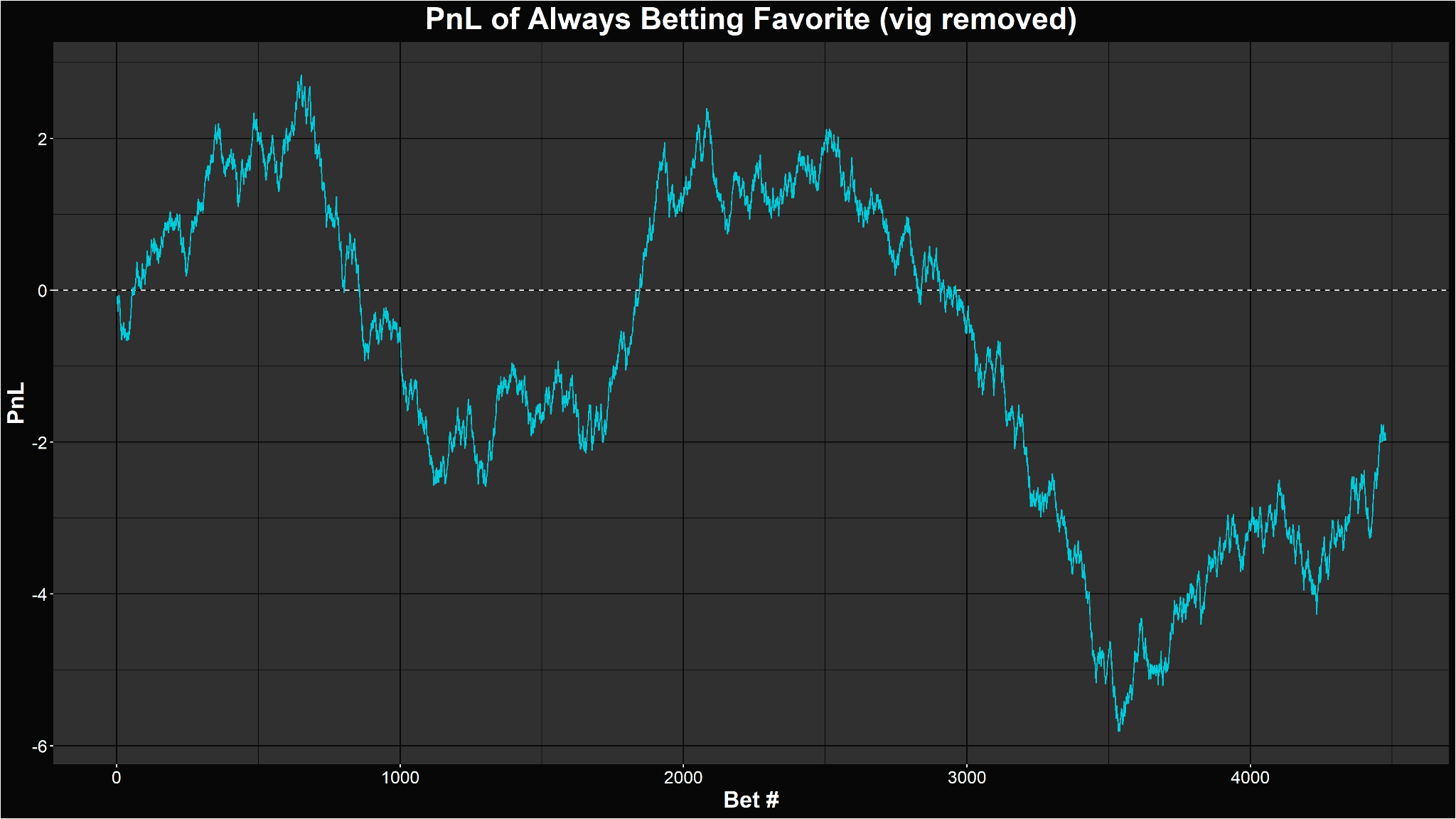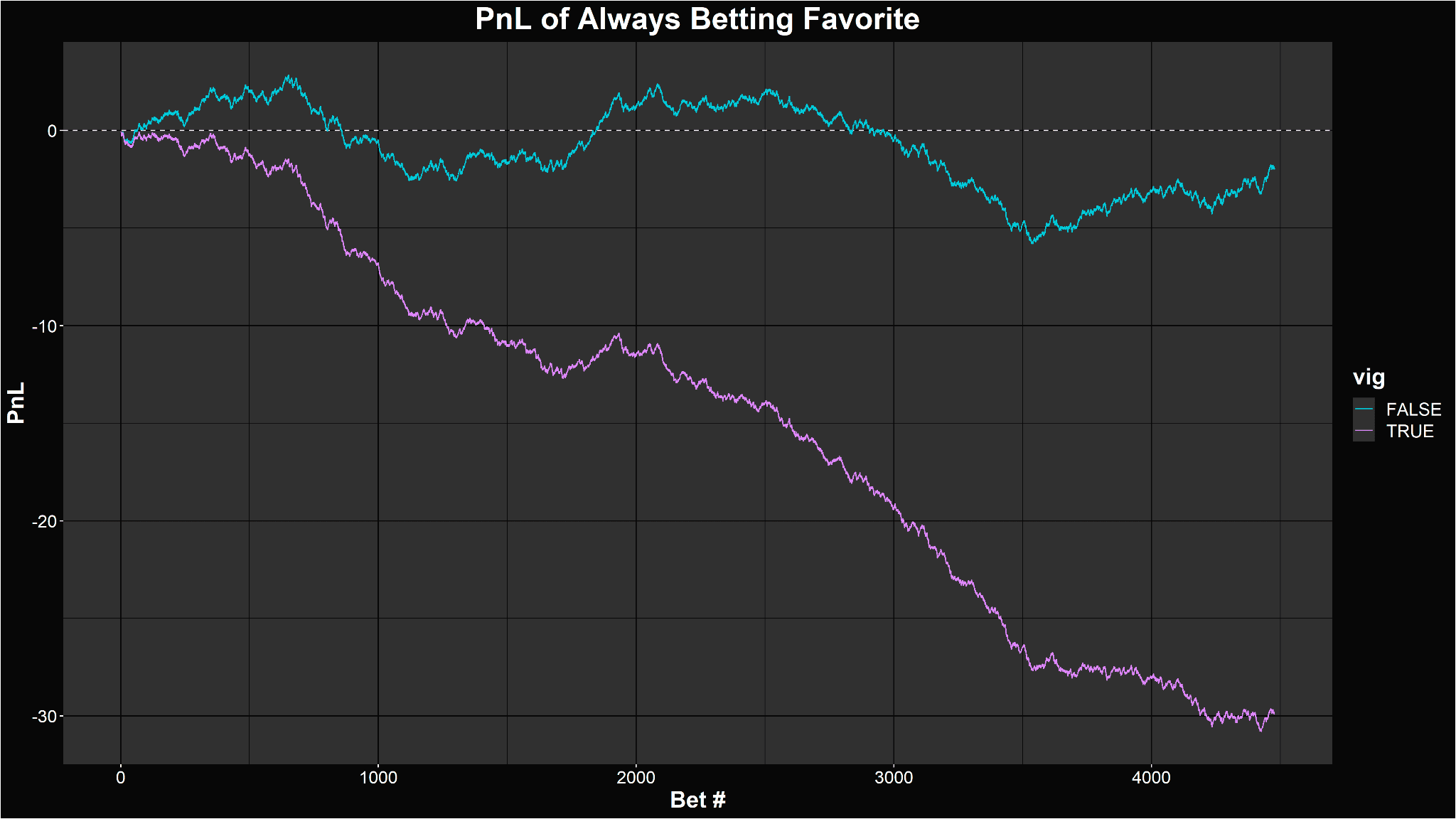XHit enter to search or ESC to closeIn our prior articles, we’ve alluded a number of times to the “vig” that an oddsmaker takes on all bets. Well, it’s finally time to drill down into exactly what it is, how to calculate it and how it affects your betting.

## What is the vig?

The vig, short for vigorish and also called the juice or cut, represents a rake that an oddsmaker takes on any bet. It helps guarantee that an oddsmaker makes a profit no matter what the outcome of an event is. As long as people are taking both sides of a bet, an oddsmaker can avoid direct exposure to an outcome and simply collect the vig as a fee for matching people up.

Let’s use a simple illustrative example: Alice and Bob want to bet on a coin flip. Alice bets \$1 on heads, and Bob bets \$1 on tails.

If they are just betting against each other, they can agree that a coin has a 50/50 chance at either outcome, and therefore the fair payout is 2.00 or +100 (depending on your preferred notation). In other words, the winner simply takes the \$1 bet of the loser.

Whatever the outcome, one of them loses \$1 and the other wins \$1, so this is a zero-sum game. Incidentally, the expected value for each of them is zero, since it’s a symmetrical bet.

What if Alice and Bob don’t know each other, but really want to bet on a coin flip? That’s where bookmakers come in. A bookmaker will help match them up. For this matching service, the bookmaker implicitly takes a fee, the vig. They won’t outright say that they’re taking some fee, however; they’ll simply adjust their odds to account for it.

Alice and Bob go to a bookmaker and are given odds of -110/-110. This means that Alice would need to bet \$110 in order to potentially win \$100, or conversely, her \$1 bet will win a profit of only 91 cents. Bob is in the same boat. Whatever happens with the coinflip, the loser will lose \$1, the winner will win 91 cents, and the bookmaker will keep the 9 cent difference.

This extra remainder is exactly the vig. It’s typically quoted as a percentage: for every two dollars bet in total (\$1 each from Alice and Bob), the house keeps 9 cents, which is 4.5%.

## The vig and implied probabilities

We ended our previous article by trying to calculate the probability of each team winning, implied from market odds:

 PuckChamp 1.25 Khan Esports 3.77

Converting these odds to win probabilities, you get:

PuckChamp: w = 1 / (p + 1) = 1 / (0.25 + 1) = 0.8 = 80%
Khan Esports: w = 1 / (p + 1) = 1 / (2.77 + 1) = 0.26525 = 26.525%

Once again, there is a vig hidden in these odds. We can calculate it the same way we did above, but it gets tricky when a bet isn’t symmetrical. Instead, there’s an easier way, which is to use the implied probabilities.

Here, PuckChamp have an 80% chance to win, while Khan Esports have a 26.525% chance to win. What does it mean that these don’t add up to 100%? Together, these two teams have a 106.525% chance to win, which sounds like nonsense. What’s really happening is that the bookmaker is paying out as if each team had a higher chance to win than it actually does, which in turn reduces the payout compared to a fair bet.

We can directly convert this into a vig: for every \$106.525 placed in bets, the bookmaker is paying out \$100. 100 / 106.525 = 93.87%, which means that the bookmaker is keeping the remaining 6.13% for itself. So, the vig is 6.13%.

We can apply the same logic to the above coin flip example. Converting -110 to a win probability yields 52.36%. Both sides of the bet add up to 104.72%, which means that for every \$104.72 of bets placed, only \$100 is being paid out. 100 / 104.72 = 95.5%, with the remaining 4.5% being the vig. This is exactly what we calculated above!

Given odds on an event, to find the vig, first convert them to implied probabilities PA and PB. The vig is simply:

vig = 1 – 1 / (PA + PB)

This works for events with more than two outcomes as well, for example matches where a draw is possible. Simply add up all of the implied probabilities for all of the possible outcomes, and use that sum as the denominator.

## Removing the vig to get normalized odds

One metric of common interest to us is what the market actually believes the odds of each team winning are. We had calculated odds of 80% and 26.525% for our two teams, and that has some meaning in terms of odds and payouts and breakevens, but it doesn’t make sense to estimate what will actually happen.

We can scale down both of those probabilities, proportionally, such that they add up to 100%. This is called normalization. Simply divide each number by the total:

PA,adjusted = PA / (PA + PB)
PB,adjusted = PB / (PA + PB)

In the above example, we would find:

PuckChamp = 80% / (80% + 26.525%) = 75.1%
Khan Esports = 26.525% / (80% + 26.525%) = 24.9%

Which now add up to 100%. This reflects the market’s true view of this match, and in fact what we’ve really done is just followed a bookmaker’s steps in reverse:

• First, they come up with a probability estimate (75.1%, 24.9%)
• Next, they add the vig, so the probabilities add up to more than 100% (80%, 26.525%)
• Finally, they convert those probabilities into odds (1.25, 3.77)

## The vig and expected value

You may recall that we had originally defined implied probability as the probability at which the expected value of a bet is zero. This can also be called the breakeven point. You’ll break even (make your money back, but no more) on your bet if you win it that percentage of your time (assuming you can repeat it infinitely).

Thus, in the above example, PuckChamp at 1.25 have a breakeven of 80%. If you win this bet 80% of the time, you should break even over time, and thus your expected value is zero:

EV = 80% * 0.25 + 20% * (-1)
EV = 0

But wait, the market actually believes that they will win only 75.1% of the time, which isn’t enough to break even! Because of the vig, the market is paying out as if PuckChamp have an 80% chance to win, while they actually only have a 75.1% chance. This means that because of the vig, you’re always below the breakeven line, and thus have negative expected value:

EV = 75.1% * 0.25 + 24.9% * (-1)
EV = -0.06125

If you believe the market’s view of probabilities, the vig will always ensure that the EV of a bet is negative.

## The vig and profits

If the vig systematically makes trades unprofitable, just how much does it eat into our profits? Let’s use a naive betting strategy, where we just put \$1 on every single match, always betting on the favorite. We’ll discuss why this is not a good idea in the next article, but for now it will serve as an illustrative example.

If the market was fair, and generally reasonably accurate in its predictions (again, more on this next time), you would generally neither make nor lose money in the long run. Here’s the PnL (profit-and-loss) plot of following this strategy in a fair market, betting on 4463 professional League of Legends matches between January 2021 and September 2021:The PnL plot of betting on 4463 professional League of Legends matches between January 2021 and September 2021. | Provided by Alacrity.gg

As expected, you stay relatively close to flat, though individual periods may have some turmoil. Now, here’s that same plot, but using the actual payouts that the market offers, with the vig added back in:The same PnL plot with the vig added back in. | Provided by Alacrity.gg

It’s a light and day difference! The vig will steadily bleed your money, and represents a significant hurdle to overcome.

## The vig and betting for value

If you should only bet when there’s positive value, and the vig ensures that expected value is always negative, doesn’t this mean that you should never bet?

There’s an important qualifier to the above statement: it is true if you believe the market’s view of probabilities. The probabilities implied by market odds tend to be reasonable, but they’re not always perfect. Value betting is built on finding matches for which you believe the market has incorrectly assessed the matchup. We’ll cover this in a future article, but this is exactly why it’s important to derive your own probabilities, independent of the market. To find value in the long term, you have to both disagree with the market, and, on average, be more correct than the market. That’s a tall ask, but it’s exactly what we’re here for.